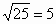# Translations of Encyclopedia about Mathematics

## Counting with Quantities

We use quantities daily with many calculations, such as with 3 kg, 30 centimetres or 4 litres. Counting with quantities is difficult only when it becomes necessary to convert one unit of measurement into another.

When counting with a greater number of quantities, it is necessary to convert them all so that they have the same unit of measurement.

Therefore: 2 kg + 150 g = 2000 g + 150 g

or 2 kg + 0,15 kg

If adding or subtracting with the same unit of measurement, we perform the operation with their numerical values and keep the units of measurement the same.

Example:

3,5 kg + 7,8 kg = 11,3 kg
8,3 m – 3,9 m = 4,4 m

When multiplying, the numerical values and units of measurements are multiplied.
If only one quantity is multiplied, then the units of measurement remain the same.

Examples:

3 m . 7 m . 8 m = 3 . 7 . 8 . m .m . m = 168 m³
3 . 5 l = 15 l

When dividing quantities, the numerical values are divided and the units of measurement are abbreviated. If the dividend is a quantity and the factor some number not equal to zero, the quotient is calculated from both values and the unit of measurement remains the same.

Examples:

15 kg : 5 kg = 3
60 sec : 15 = 4 sec

Translating Spanish Portuguese Translations Portuguese Spanish Translating Hungarian Dutch Translations Dutch Hungarian Translating Polish

## Terms

A term is a sort of expression, composed of numbers, variables and operators (such as +, -, * etc.) in a particular order. When we add, subtract, divide or multiply two expressions, we get yet another expression. The result of exponentiating or extraction of root of some terms once again gives a term, or expression.

Some examples of terms without variables:

1, 7, 87, 4+2, 8 I 5

Examples of expressions with variables:

X, y, a, 5x, 14a, x Ì y, 5x + a

Both of the following examples are expressions which relate to an equation or inequality, which we call conclusions.

X = 2, 7x + 2 < 27

Calculating with Expressions

When calculating with expressions, the same rules apply like when calculating with normal numbers.

8 + 2 = 10
(11 + 3) : 7 = 2etc.

When calculating with expressions which contain variables, it is necessary to adhere to the following rules:

1. When adding or subtracting two or more different variables, the following applies:

x + y – z = x + y – z

Therefore, the expression cannot be shortened or simplified by contraction.

1. When adding or subtracting two or more of the same variables, the following applies:

X + 8x + 17x – 5x = 21x

Multiples of the variable x can be added, as such shortening the expression.

2. When multiplying two or more different variables, the following applies:

5x . 7y = 5 . 7 . x . y = 35xy

The expression can be expressed by composite.

3. When multiplying two or more of the same variables, the following applies:

3z . 8z = 3 . 8 . z . z = 24 z²

This expression can be consolidated as well.

When dividing, the same rules apply and the expression is called a fractional expression, in which case the nominators and denominators can be reduced to their lowest term.

Because a denominator of a fraction can never have a value of zero, the division of terms in a denominator must consider the definition of its variables, where these variable can never attain such a value to give the denominator a value of zero.

With the variable x in the expression 3x + 2, its definition can be the set of real numbers R (expressed in mathematics as x Î R).

For example, the variable y in the expression 12:(7-y) cannot have the value of 7 because that would give the denominator a value of zero. For this reason, the definition of the variable y is the set of all real numbers excluding the value of 7 (or: yÎ R\(7) ).

For y = 1 :

12 : (7 – 1) = 2

For y = 7:

12 : (7 – 7) = undefined.

In this case, the value of 7 must not be included in the definition of variable y because that would give the denominator a value of zero.

Translating Russian Ukrainian Translations Ukrainian Russian Translating Persian Arab Translations Arab Persian Translating TurkishEnter your search terms Submit search form